## 09 Oscillations

<insert some write up on Oscillations>

## Mass and Spring in Gravity HTML5 JavaScript Simulation by Darren Z Tan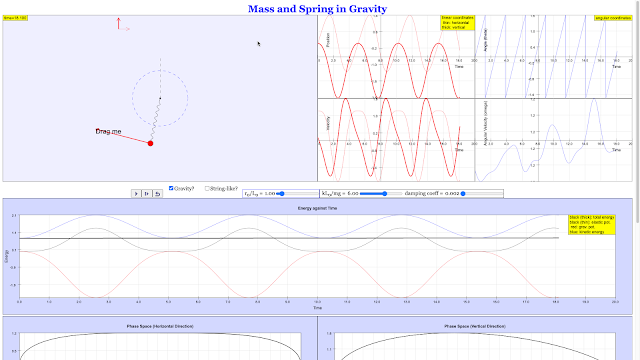## SHM Bungee Displacement against Time Graph with Damping HTML5 Applet Javascript1.5 1 1 1 1 1 1 1 1 1 1 Rating 1.50 (1 Vote)

## SHM Bungee Phase Circle & Damping HTML5 Applet Javascript## SHM Bungee Displacement vs Velocity Graph HTML5 Applet Javascript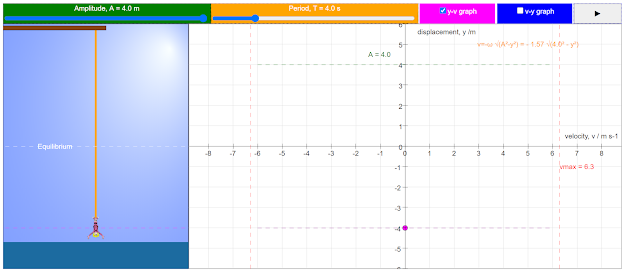## SHM Bungee Displacement-Time Graph HTML5 Applet Javascript## SHM Bungee with Damping HTML5 Applet Javascript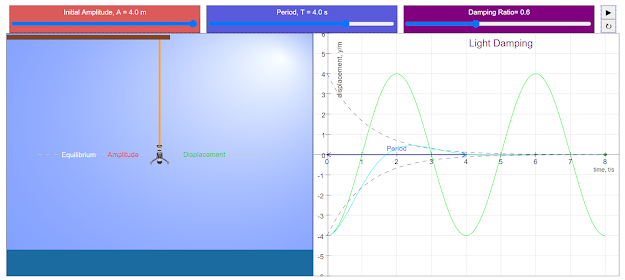## SHM Bungee Acceleration vs Displacement Graph HTML5 Applet Javascript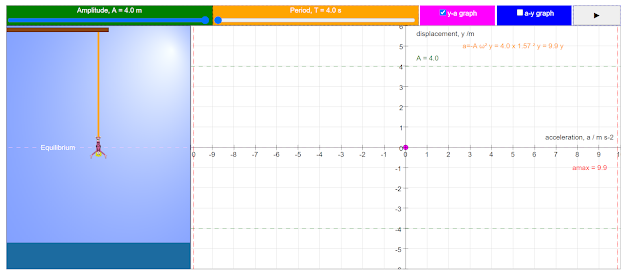## SHM Overall Bungee HTML5 Applet Javascript## SHM Bungee Phase Circle Graph HTML5 Applet Javascript## SHM Bungee Phase Graph HTML5 Applet Javascript## SHM Bungee SVA Graph HTML5 Applet Javascript## Horizontal Spring SVA Graph HTML5 Applet Javascript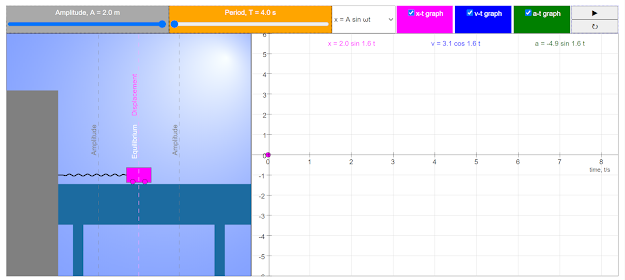## PICUP Harmonic and Anharmonic Oscillations of a Boat JavaScript Simulation Applet HTML5## PICUP Plane Rigid Pendulum JavaScript Simulation Applet HTML5## PICUP Hanging Simple Harmonic Oscillator JavaScript Simulation Applet HTML5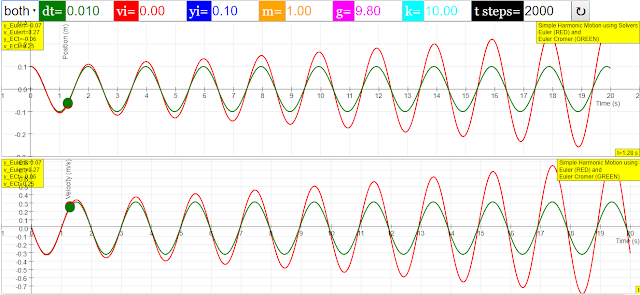## Three Independent Spring Systems JavaScript Simulation Applet HTML5## Student Learning Space Force Oscillation JavaScript HTML5 Applet Simulation Model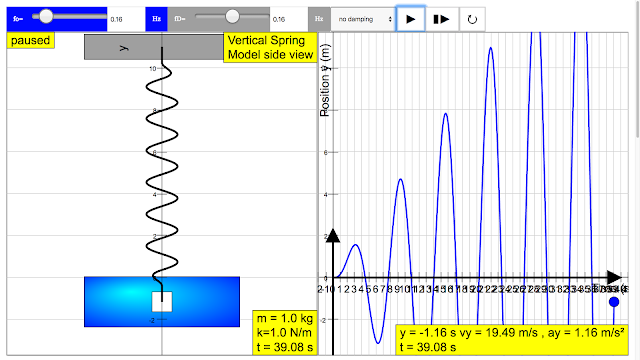## Student Learning Space Resonance type 0 HTML5 Applet Simulation Model## Student Learning Space Resonance type 1 HTML5 Applet Simulation Model## Student Learning System Degree of Damping Ammeter Example HTML5 Applet Simulation Model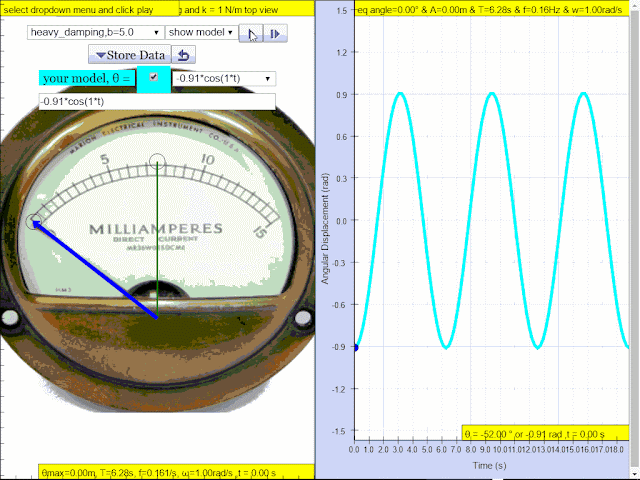## Student Learning Space Damping Car HTML5 Applet Simulation Model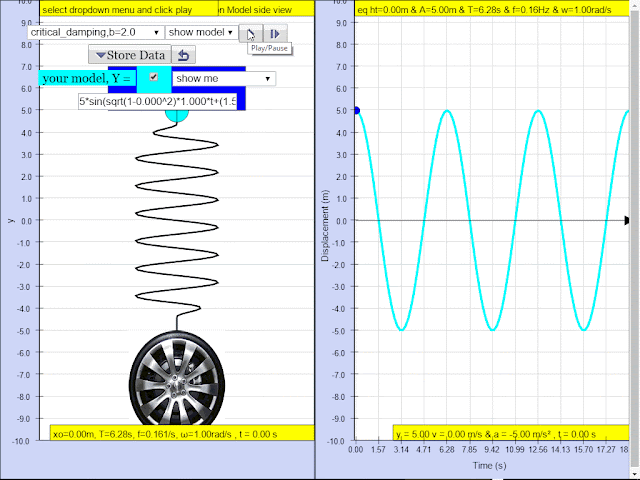## Tracker SHM 350g Initial Extension Tension Weight ModelRating 5.00 (1 Vote)

## Tracker SHM 300g Tension Weight Model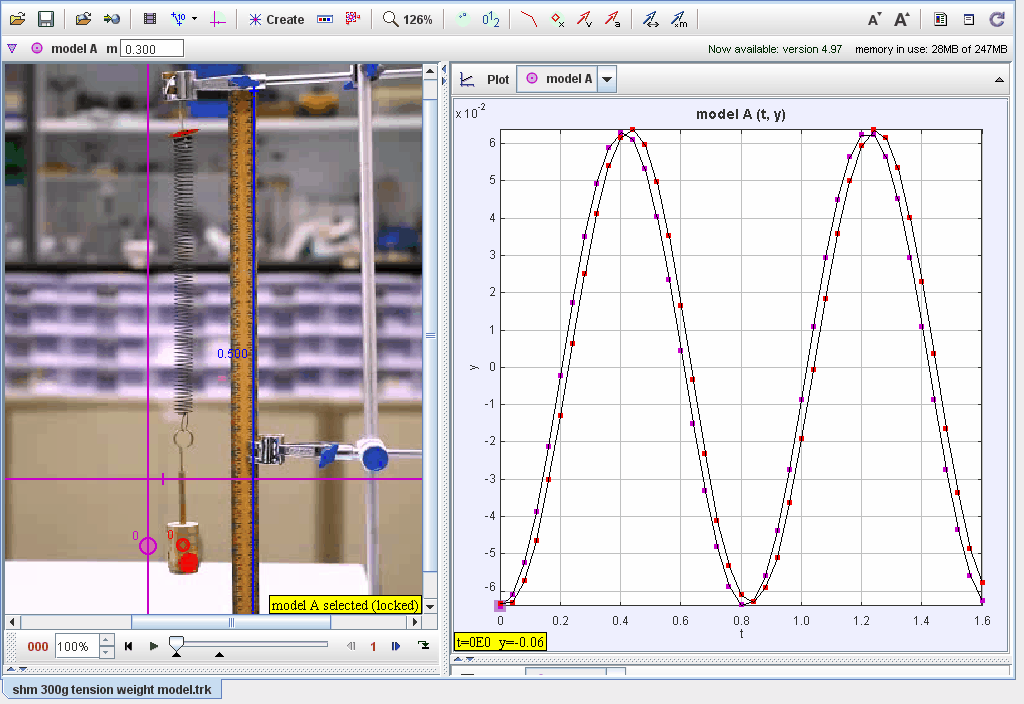Rating 5.00 (1 Vote)

## Tracker SHM 200g Tension Weight Model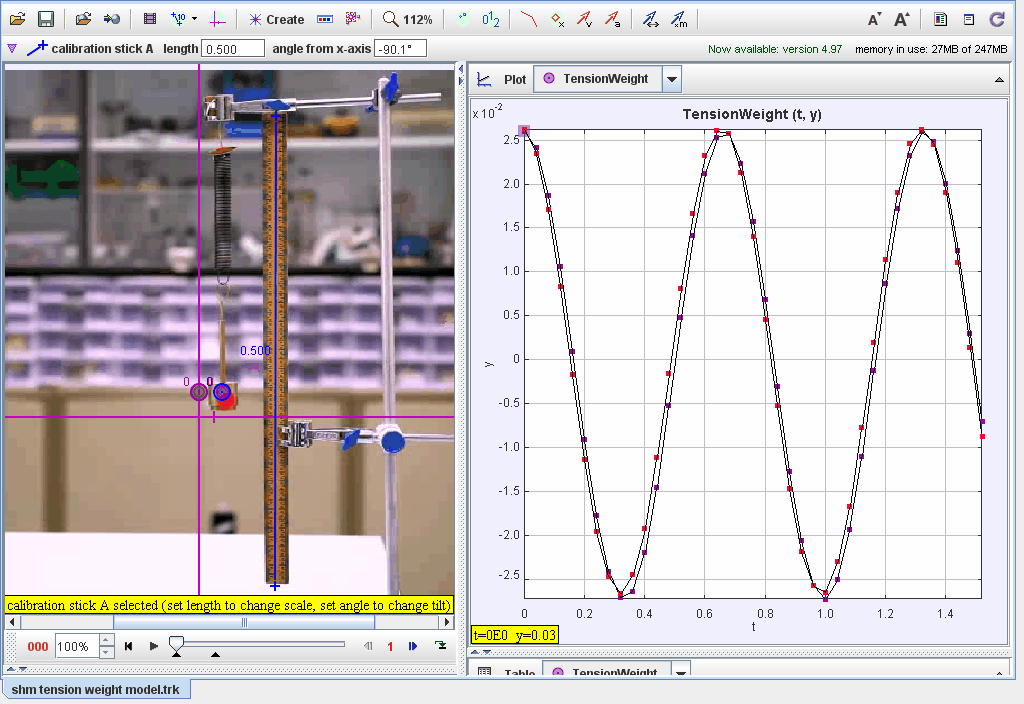Rating 5.00 (1 Vote)

## epub3 e-Book on SHM Simple Harmonic Motion4.9 1 1 1 1 1 1 1 1 1 1 Rating 4.90 (5 Votes)

## 10 Pendulum JavaScript Model Simulation Applet HTML5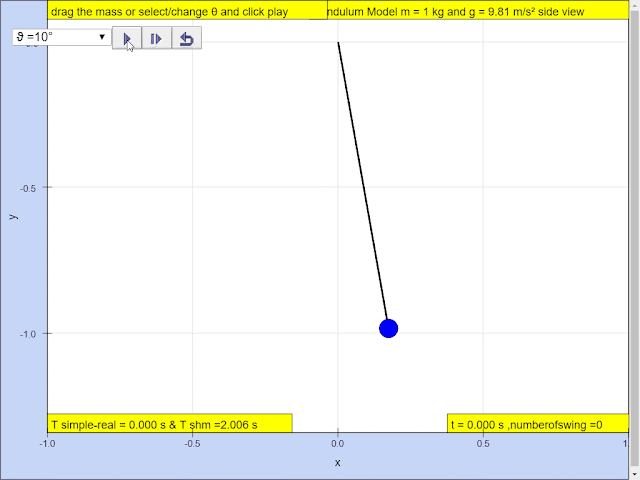## Amazing Pendulum Wave Effect JavaScript Model Simulation Applet HTML5Rating 5.00 (1 Vote)

## Real Time Evolution Line Code 10 Pendulum JavaScript Model Simulation Applet HTML5## Real Time ODE 10 Pendulum JavaScript Model Simulation Applet HTML5## 10.1.3 Horizontal Spring Mass Model X vs t JavaScript HTML5 Applet Simulation Model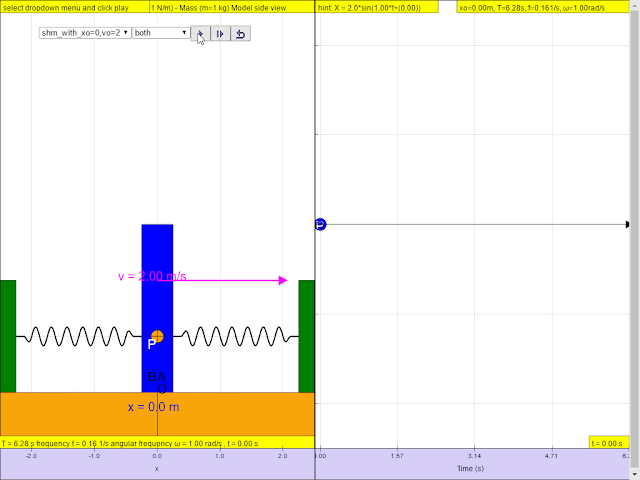4.9 1 1 1 1 1 1 1 1 1 1 Rating 4.90 (5 Votes)

## 10.1.3.2.3 Circular Motion Model and Relationship to Simple Harmonic Motion JavaScript HTML5 Applet Simulation Model## 10.2 Definition of Simple Harmonic Motion and Horizontal Spring Mass Model A vs X JavaScript HTML5 Applet Simulation Model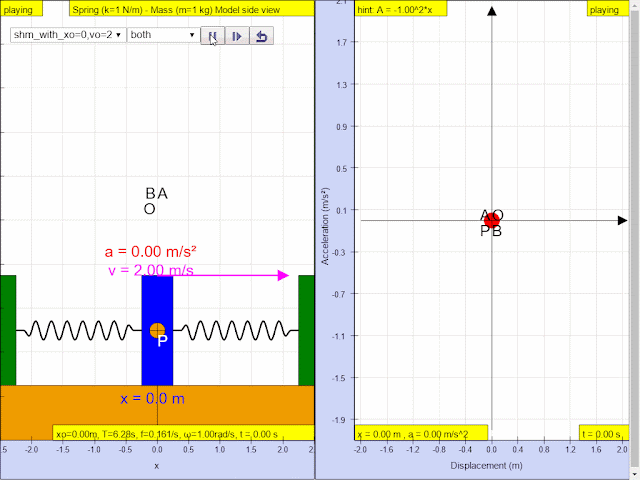## 10.2.2 Defining Equation of Simple Harmonic Motion and Horizontal Spring Mass Model A vs T## 10.2.3 Horizontal Spring Mass Model Velocity versus Position JavaScript HTML5 Applet Simulation Model## 10.2.4 Horizontal Spring Mass Model Acceleration versus Position JavaScript HTML5 Applet Simulation Model## 10.2.5 Example Spring (k=9 N/m) - Mass (m=1 kg) model x0=0, v0 =6 Position versus time JavaScript HTML5 Applet Simulation Model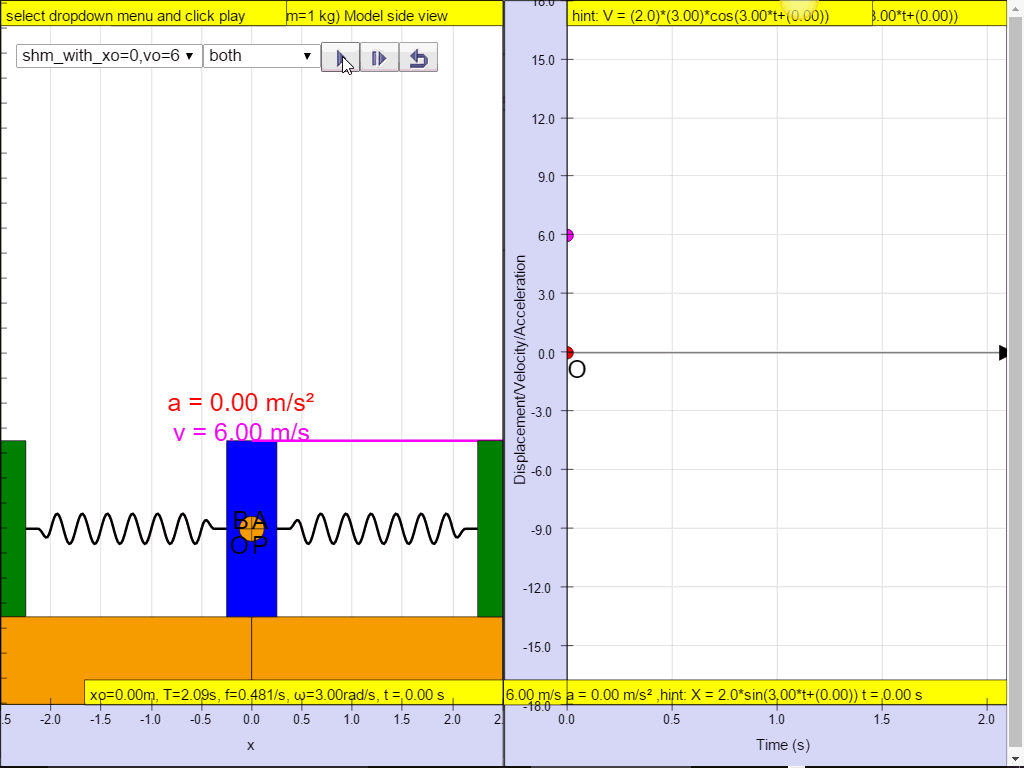## 10.2.6 Example Spring (k=9 N/m) - Mass (m=1 kg) JavaScript HTML5 Applet Simulation Model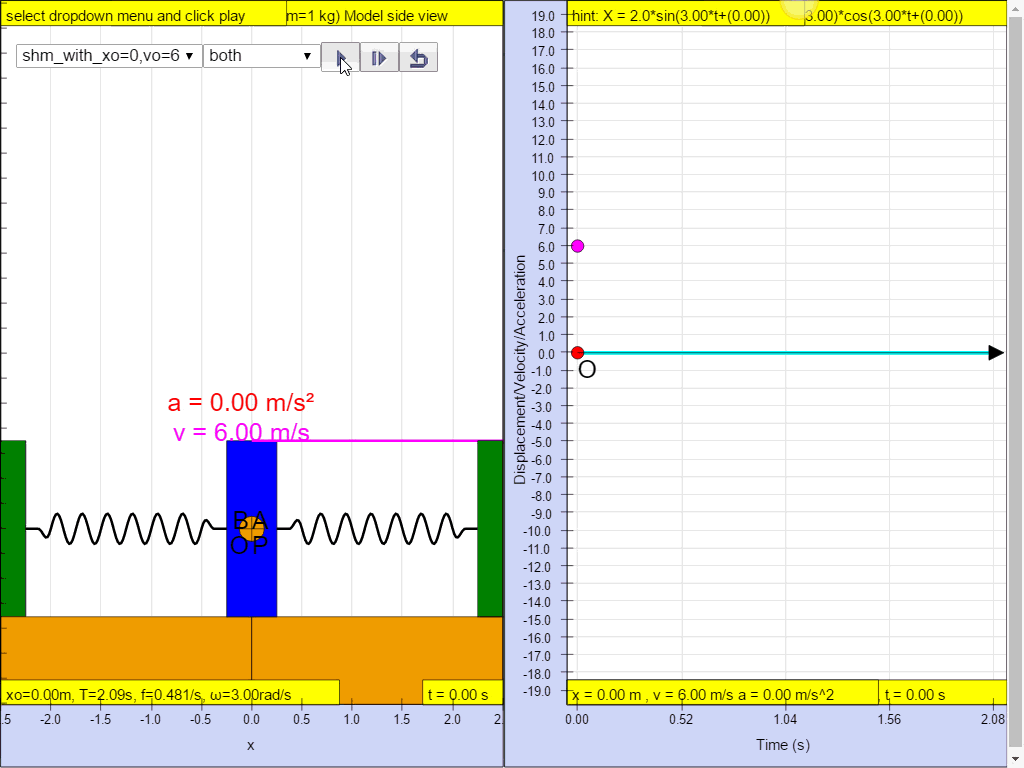## 10.2.7 Example Spring k=(π^2)/9 N/m, mass m=1 kg model versus Time for t = 1.5 Period JavaScript HTML5 Applet Simulation Model## 10.2.8 Example Spring k=(π^2)/25 N/m) mass m=1 kg model versus Time JavaScript HTML5 Applet Simulation Model## 10.2.9 Example Spring (k=(π^2)/4 N/m) - Mass (m=1 kg) model versus Time## 10.2.10 Example Spring (k=16 N/m) - Mass (m=1 kg) model Velocity versus Position JavaScript HTML5 Applet Simulation Model## 10.2.11 Example Vertical Spring Mass JavaScript HTML5 Applet Simulation Model## 10.2.12 Example Vertical Spring Mass Model Position y = 7m JavaScript HTML5 Applet Simulation Model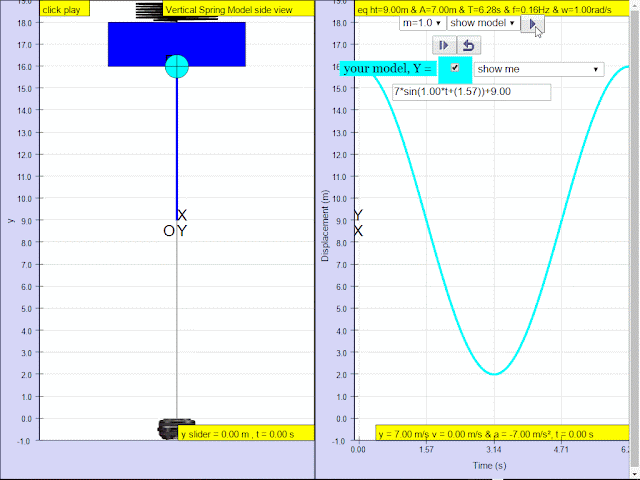## 10.2.13 Experimental Investigation of Vertical Oscillator Model Position y = 2 JavaScript HTML5 Applet Simulation Model## 10.3 Variation with Time of Energy in Simple Harmonic Motion JavaScript HTML5 Applet Simulation Model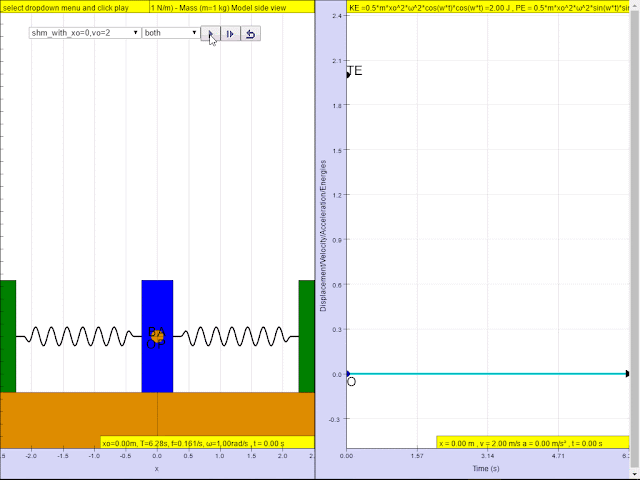## 10.4(b) Variation with displacement of energy in simple harmonic motion JavaScript HTML5 Applet Simulation Model## 10.4.3 Example JavaScript HTML5 Applet Simulation Model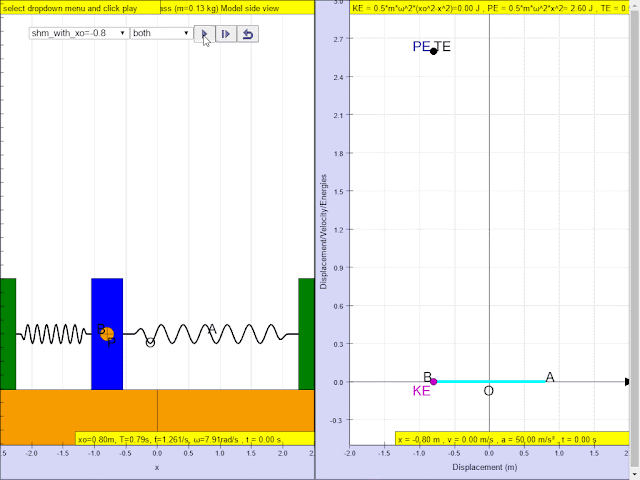## 10.5 Example of investigation of motion of an oscillator using experimental and graphical method HTML5 Applet Simulation Model